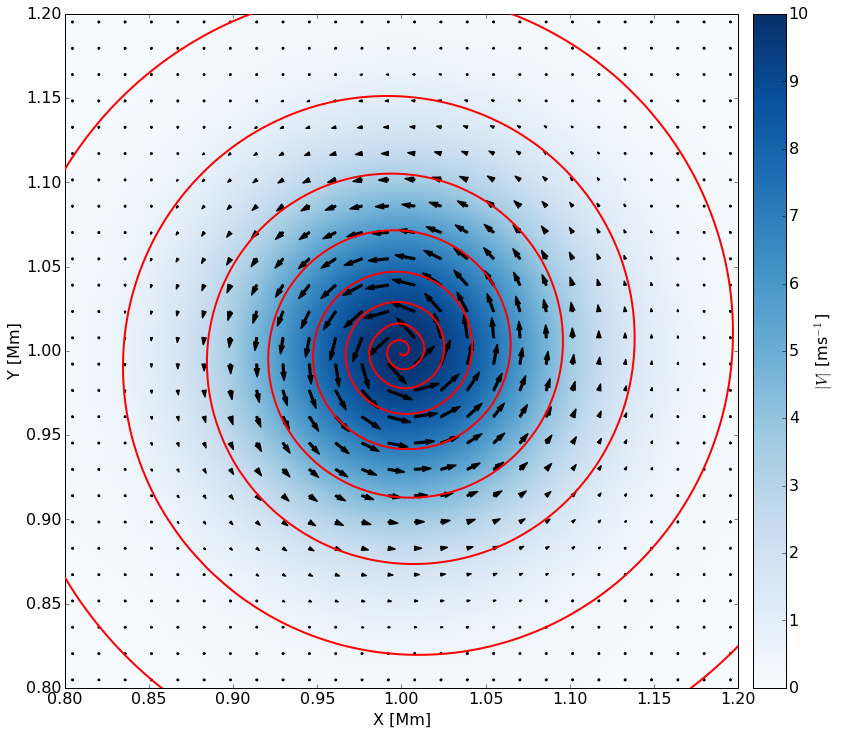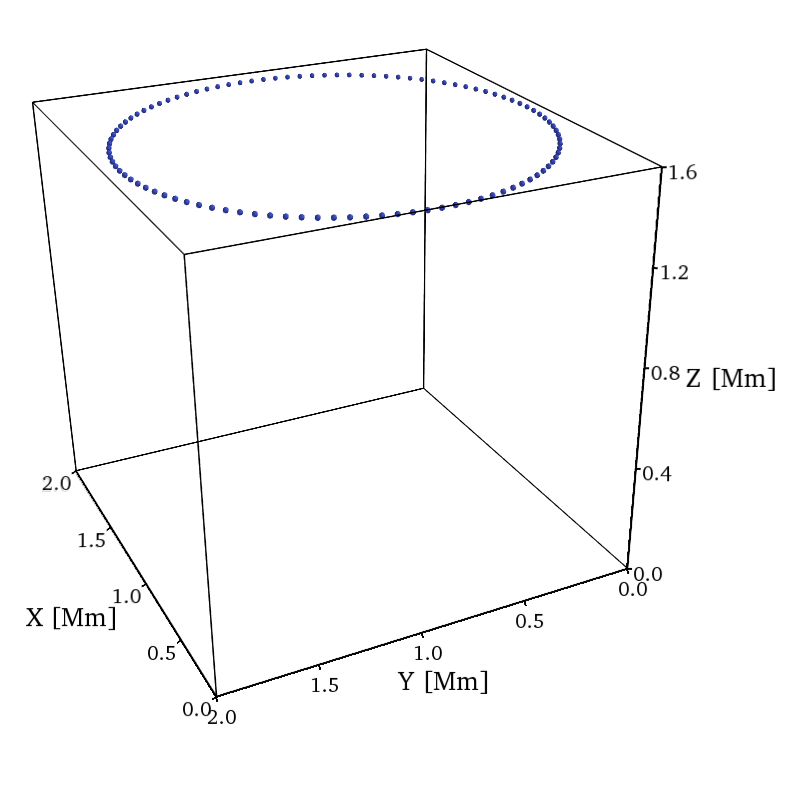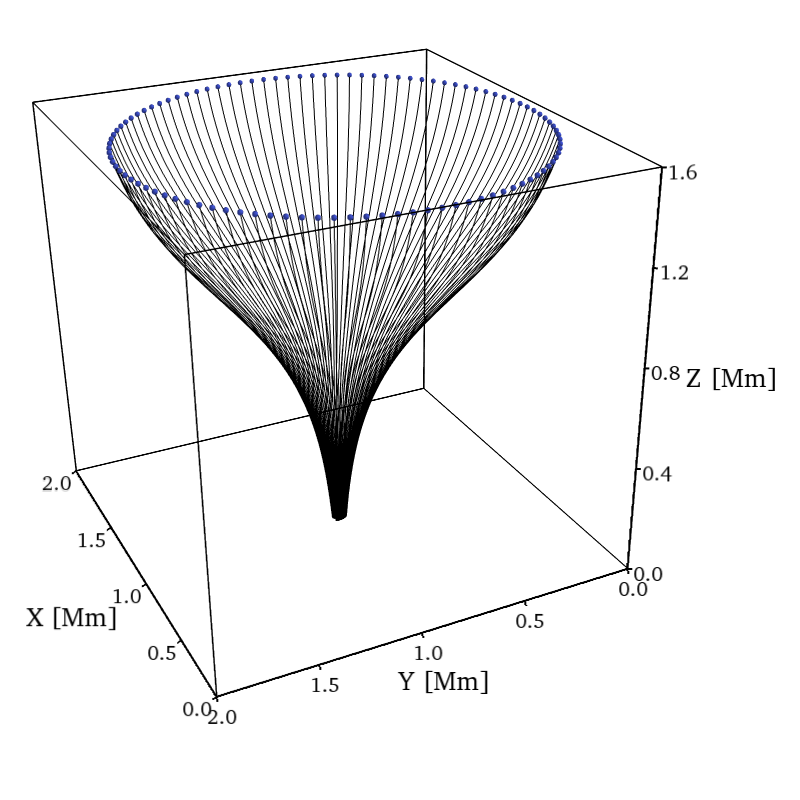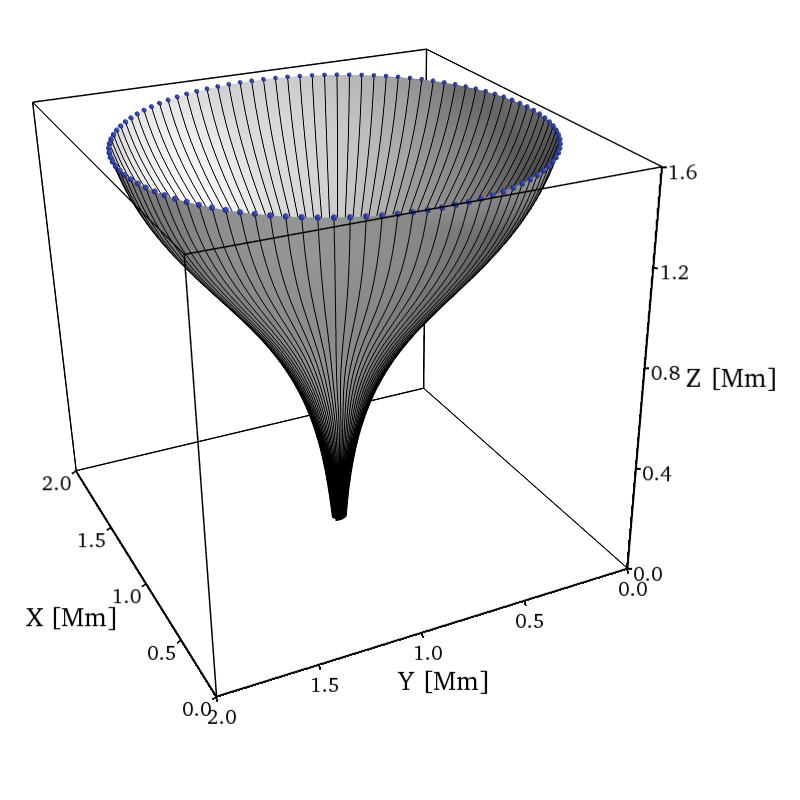# Simulating the Generation of MHD Waves in the Low-Solar Atmosphere

Stuart J. Mumford, Robertus Erdélyi

Solar Physics & Space Plasma Research Centre (SP2RC), School of Mathematics and Statistics, The University of Sheffield# The Sun is too Hot!

Specifically the outer layers of the solar atmosphere, the corona, temperatures reach millions of degrees Kelvin.

### The coronal heating problem:

How does the energy get from the surface of the Sun to the corona?

What energy transport mechanism transfers the energy?# MHD Wave Heating of the Solar Corona

MHD Waves are considered to be a good candidate for contributuing to coronal heating. Here we look at the generation of these waves in the photosphere.

Under idealised conditions and geometry there are three MHD wave modes:

1. Fast
2. Slow
3. Alfvén

Under more complex conditions there are many more modes, harmonics and other effects. Different wave modes have different potential for depositing their energy in the upper atmosphere, they are also excited by different driving motions.

## The Code

The Sheffield Advanced Code (SAC) is a fully non-linear 1-3D MHD and HD code.

It seperates out the static background conditions from the pertubation components, making it easier to simulate the large variation in scales and to easily isolate the effects of wave pertubations.

# Our Simulation Domain

A hydrodynamic background with a magnetic field added to the top and then magnetic pressure subtracted.# Driving Waves

To study waves in the simulations we must excite them. This means adding in a velocity field at the base of the simulation to replicate the turblaent motion of the photosphere.

# Logarithmic Spiral Driver

In :
import numpy as np
from streamlines import Streamlines
import matplotlib as mpl
%matplotlib inline
mpl.rc('font', size=16.0)
import matplotlib.pyplot as plt
from mpl_toolkits.axes_grid1 import make_axes_locatable
#Use Equation 1 to calculate the vector field in a 2D plane to plot it.
time = np.linspace(0,60,480)
dt = time[1:] - time [:-1]
period = 240.

x = np.linspace(7812.5,1992187.5,128)
y = np.linspace(7812.5,1992187.5,128)

x_max = x.max()
y_max = y.max()

xc = 1.0e6
yc = 1.0e6

xn = x - xc
yn = y - yc

delta_x=0.1e6
delta_y=0.1e6

xx, yy = np.meshgrid(xn,yn)
exp_y = np.exp(-(yn**2.0/delta_y**2.0))
exp_x = np.exp(-(xn**2.0/delta_x**2.0))

exp_x2, exp_y2= np.meshgrid(exp_x,exp_y)
exp_xyz = exp_x2 * exp_y2

#==============================================================================
# Define Driver Equations and Parameters
#==============================================================================
#A is the amplitude, B is the spiral expansion factor
A = 10

#Tdamp defines the damping of the driver with time, Tdep is the ocillator
tdamp = lambda time1: 1.0 #*np.exp(-(time1/(period)))
tdep = lambda time1: np.sin((time1*2.0*np.pi)/period) * tdamp(time1)

#Define a peak index to use for scaling in the inital frame
max_ind = np.argmax(tdep(time) > 0.9998)

#Logarithmic
B = 0.05
phi = np.arctan2(1,B)
theta = np.arctan2(yy,xx)

uy = np.sin(theta + phi)
ux =  np.cos(theta + phi)

vx = lambda time1: (ux / np.sqrt(ux**2 + uy**2)) * exp_xyz * tdep(time1) * A
vy = lambda time1: (uy / np.sqrt(ux**2 + uy**2)) * exp_xyz * tdep(time1) * A

vv = np.sqrt(vx(time[max_ind])**2 + vy(time[max_ind])**2)

fig, ax = plt.subplots(1,1, figsize=(12,12))

#============================================================================
# Do the Plotting
#============================================================================
# Calculate Streamline
slines = Streamlines(x,y,vx(time[max_ind]),vy(time[max_ind]),maxLen=7000,
x0=xc, y0=yc, direction='forwards')

im = ax.imshow(vv, cmap='Blues', extent=[7812.5,x_max,7812.5,y_max])
im.set_norm(mpl.colors.Normalize(vmin=0,vmax=10))
#ax.hold()

Sline, = ax.plot(slines.streamlines,slines.streamlines,color='red',linewidth=2, zorder=40)

divider = make_axes_locatable(ax)
cbar = plt.colorbar(im,cax)
cbar.set_label(r"$|V|$ [ms$^{-1}$]")
scalar = mpl.ticker.ScalarFormatter(useMathText=False,useOffset=False)
scalar.set_powerlimits((-3,3))
cbar.formatter = scalar
cbar.ax.yaxis.get_offset_text().set_visible(True)
cbar.update_ticks()
#cbar.solids.set_rasterized(True)
cbar.solids.set_edgecolor("face")

qu = ax.quiver(x,y,vx(time[max_ind]),vy(time[max_ind]),scale=25*A,color='k',zorder=20, linewidth=1.5)
ax.axis([8.0e5,12.0e5,8.0e5,12.0e5])

ax.xaxis.set_major_formatter(scalar)
ax.yaxis.set_major_formatter(scalar)
ax.xaxis.get_offset_text().set_visible(False)
ax.yaxis.get_offset_text().set_visible(False)
ax.set_xlabel("X [Mm]")
ax.set_ylabel("Y [Mm]")

plt.tight_layout()## Different Periods

We drive the simulation with different period logarithmic sprial drivers, changing the amplitude to input the same kinetic energy. $$A^2 \propto \frac{1}{P}$$

Period [seconds] Amplitude [ms$^{-1}$]
$30.0$ $20\sqrt{2}$
$60.0$ $20$
$90.0$ $20\sqrt{\frac{2}{3}}$
$120.0$ $10\sqrt{2}$
$150.0$ $4\sqrt{10}$
$180.0$ $\frac{20}{\sqrt{3}}$
$210.0$ $20\sqrt{\frac{2}{7}}$
$240.0$ $10$
$270.0$ $\frac{20}{3}\sqrt{2}$
$300.0$ $4\sqrt{5}$

# Analysis

Again, under very idealised conditions they seperate into the components of the wave that are perpendicular, parallel and azimuthal to the magnetic field.

Under not so idealised conditions, how do we extract these components?

In :
surf_seeds = ttf.make_circle_seeds(100, 60, **domain)
seeds = np.array(surf_seeds.points)

seed_points = mlab.points3d(seeds[:,0], seeds[:,1], seeds[:,2],
color=(0.231, 0.298, 0.752), scale_mode='none',
scale_factor=1.5)

mlab_view(fig.scene)

Out:In :
mlab_view(fig.scene)

Out:In :
flux_surface = mlab.pipeline.surface(surface.output)
flux_surface.actor.mapper.scalar_visibility = False
flux_surface.actor.property.color = (0.8,0.8,0.8)
flux_surface.actor.property.line_width = 0.5
mlab_view(fig.scene)

Out:In :
axes.visible = False
outline.visible = False
flux_surface.actor.property.edge_visibility = True
mlab_view(fig.scene, azimuth = 90, elevation = 75, distance=80, focalpoint=[63, 120, 110], aa=20)

Out: